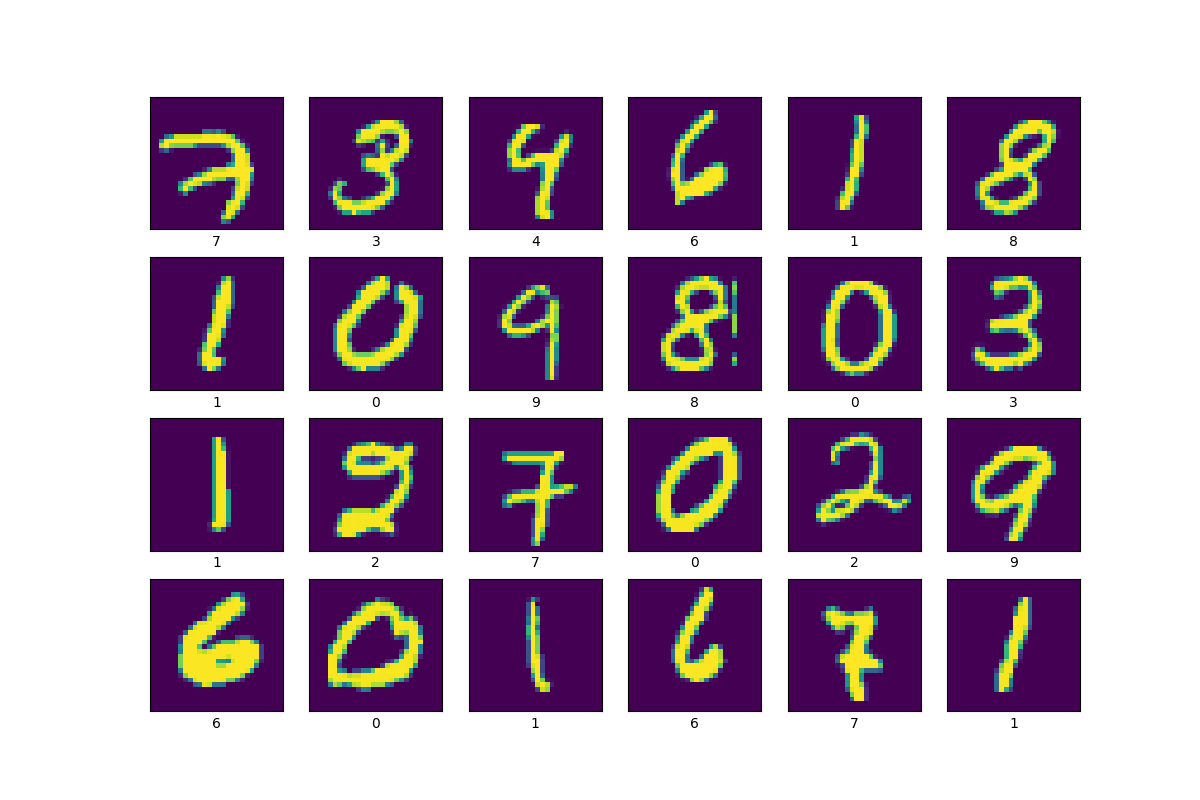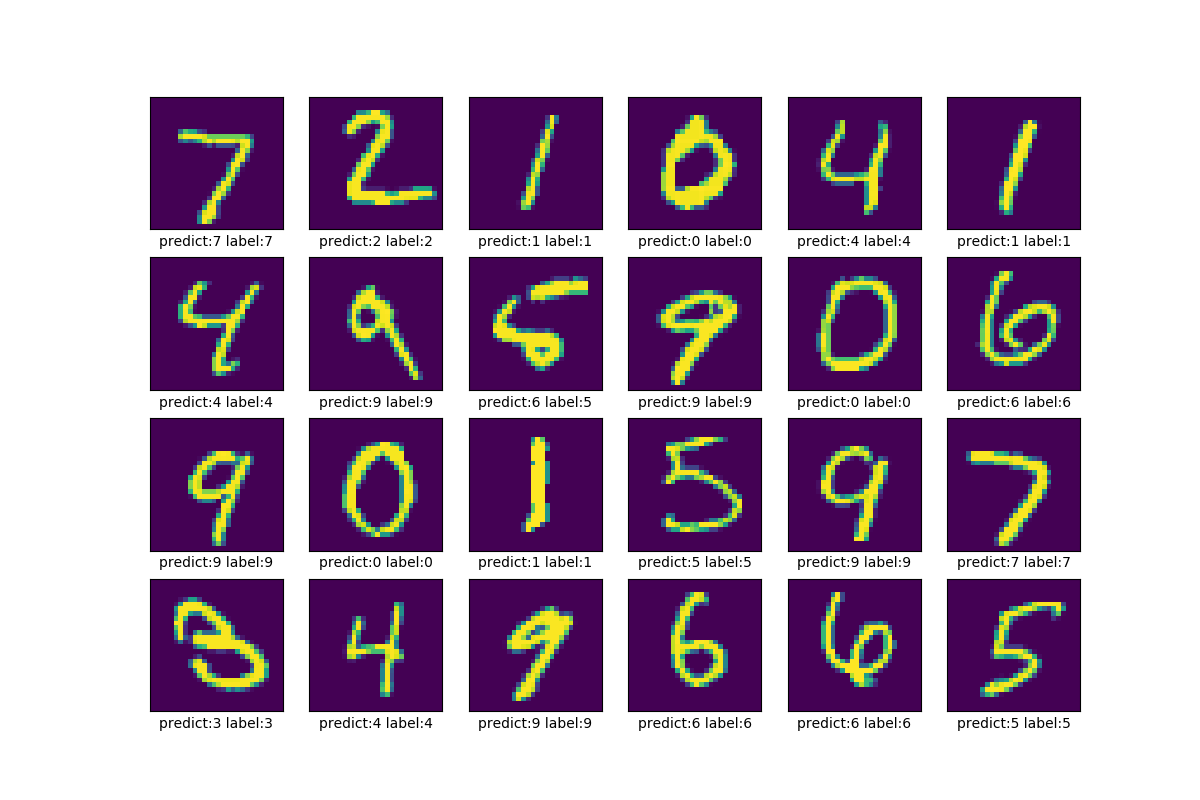# TensorFlow从1到2（二）#### 图片样本可视化

MNIST的样本数据来自Yann LeCun的项目网站。如果网速比较慢的话，可以先用下载工具下载，然后放置到自己设置的数据目录，比如工作目录下的data文件夹，input_data检测到已有数据的话，不会重复下载。

``````#!/usr/bin/env python3

# 引入mnist数据预读准备库
# 此处为了同以前的讲解对比，沿用之前的引用文件
import input_data
# tensorflow 2.0库
import tensorflow as tf
# 引入绘图库
import matplotlib.pyplot as plt

# 这里使用mnist数据预读准备库检查给定路径是已经有样本数据，
# 没有的话去网上下载，并保存在指定目录
# 已经下载了数据的话，将数据读入内存，保存到mnist对象中

# 样本集的结构如下：
# mnist.train 训练数据集
# mnist.validation 验证数据集
# mnist.test 测试数据集
# len(mnist.train.images)=55000
# len(mnist.train.images)=784
# len(mnist.train.labels)=10

def plot_image(i, imgs, labels):
# 将1维的0-1的数据转换为标准的0-255的整数数据，2维28x28的图片
image = tf.floor(256.0 * tf.reshape(imgs[i], [28, 28]))
# 原数据为float，转换为uint8字节数据
image = tf.cast(image, dtype=tf.uint8)
# 标签样本为10个字节的数组，为1的元素下标就是样本的标签值
# 这里使用argmax方法直接转换为0-9的整数
label = tf.argmax(labels[i])
plt.grid(False)
plt.xticks([])
plt.yticks([])
# 绘制样本图
plt.imshow(image)
# 显示标签值
plt.xlabel("{}".format(label))

def show_images(num_rows, num_cols, images, labels):
num_images = num_rows*num_cols
plt.figure('Train Samples', figsize=(2*num_cols, 2*num_rows))
# 循环显示前num_rows*num_cols副样本图片
for i in range(num_images):
plt.subplot(num_rows, num_cols, i+1)
plot_image(i, images, labels)
plt.show()

# 显示前4*6=24副训练集样本
show_images(4, 6, mnist.train.images, mnist.train.labels)
``````#### TensorFlow 2.0中的模型构建

``````#!/usr/bin/env python
# -*- coding=UTF-8 -*-

import input_data

import tensorflow as tf
sess = tf.InteractiveSession()

#对W/b做初始化有利于防止算法陷入局部最优解，
#文档上讲是为了打破对称性和防止0梯度及神经元节点恒为0等问题，数学原理是类似问题
#这两个初始化单独定义成子程序是因为多层神经网络会有多次调用
def weight_variable(shape):
#填充“权重”矩阵，其中的元素符合截断正态分布
#可以有参数mean表示指定均值及stddev指定标准差
initial = tf.truncated_normal(shape, stddev=0.1)
return tf.Variable(initial)
def bias_variable(shape):
#用0.1常量填充“偏移量”矩阵
initial = tf.constant(0.1, shape=shape)
return tf.Variable(initial)

#定义占位符，相当于tensorFlow的运行参数，
#x是输入的图片矩阵，y_是给定的标注标签，有标注一定是监督学习
x = tf.placeholder("float", shape=[None, 784])
y_ = tf.placeholder("float", shape=[None, 10])

#定义输入层神经网络，有784个节点，1024个输出，
#输出的数量是自己定义的，要跟第二层节点的数量吻合
W1 = weight_variable([784, 1024])
b1 = bias_variable()
#使用relu算法的激活函数，后面的公式跟前一个例子相同
h1 = tf.nn.relu(tf.matmul(x, W1) + b1)

#定义第二层（隐藏层）网络，1024输入，512输出
W2 = weight_variable([1024, 512])
b2 = bias_variable()
h2 = tf.nn.relu(tf.matmul(h1, W2) + b2)

#定义第三层（输出层），512输入，10输出，10也是我们希望的分类数量
W3 = weight_variable([512, 10])
b3 = bias_variable()
#最后一层的输出同样用softmax分类（也算是激活函数吧）
y3=tf.nn.softmax(tf.matmul(h2, W3) + b3)

#交叉熵代价函数
cross_entropy = -tf.reduce_sum(y_*tf.log(y3))
#计算正确率以评估效果
correct_prediction = tf.equal(tf.argmax(y3,1), tf.argmax(y_,1))
accuracy = tf.reduce_mean(tf.cast(correct_prediction, "float"))
#tf初始化及所有变量初始化
sess.run(tf.global_variables_initializer())
#进行20000步的训练
for i in range(20000):
#每批数据50组
batch = mnist.train.next_batch(50)
#每100步进行一次正确率计算并显示中间结果
if i%100 == 0:
train_accuracy = accuracy.eval(feed_dict={
x:batch, y_: batch})
print "step %d, training accuracy %g"%(i, train_accuracy)
#使用数据集进行训练
train_step.run(feed_dict={x: batch, y_: batch})

#完成模型训练给出最终的评估结果
print "test accuracy %g"%accuracy.eval(feed_dict={
x: mnist.test.images, y_: mnist.test.labels})
``````

• 使用了一个三层的神经网络，每一层都使用重复性的代码构建
• 每一层的代码中，要精心计算输入和输出数据的格式、维度，使得每一层同上、下两层完全吻合
• 精心设计损失函数（代价函数）和选择回归算法
• 复杂的训练循环

``````#!/usr/bin/env python3

# 引入mnist数据预读准备库
# 此处为了同以前的讲解对比，沿用之前的引用文件
import input_data
# tensorflow库
import tensorflow as tf
# tensorflow 已经内置了keras
from tensorflow import keras
# 引入绘图库
import matplotlib.pyplot as plt

# 这里使用mnist数据预读准备库检查给定路径是已经有样本数据，
# 没有的话去网上下载，并保存在指定目录
# 已经下载了数据的话，将数据读入内存，保存到mnist对象中

# 样本集的结构如下：
# mnist.train 训练数据集
# mnist.validation 验证数据集
# mnist.test 测试数据集
# len(mnist.train.images)=55000
# len(mnist.train.images)=784
# len(mnist.train.labels)=10

def plot_image(i, imgs, labels, predictions):
# 将1维的0-1的数据转换为标准的0-255的整数数据，2维28x28的图片
image = tf.floor(256.0 * tf.reshape(imgs[i], [28, 28]))
# 原数据为float，转换为uint8字节数据
image = tf.cast(image, dtype=tf.uint8)
# 标签样本为10个字节的数组，为1的元素下标就是样本的标签值
# 这里使用argmax方法直接转换为0-9的整数
label = tf.argmax(labels[i])
prediction = tf.argmax(predictions[i])
plt.grid(False)
plt.xticks([])
plt.yticks([])
# 绘制样本图
plt.imshow(image)
# 显示标签值，对比显示预测值和实际标签值
plt.xlabel("predict:{} label:{}".format(prediction, label))

def show_images(num_rows, num_cols, images, labels, predictions):
num_images = num_rows*num_cols
plt.figure('Predict Samples', figsize=(2*num_cols, 2*num_rows))
# 循环显示前num_rows*num_cols副样本图片
for i in range(num_images):
plt.subplot(num_rows, num_cols, i+1)
plot_image(i, images, labels, predictions)
plt.show()

# 原文中已经说明了，当前是10个元素数组表示一个数字，
# 值为1的那一元素的索引就是代表的数字，这是分类算法决定的
# 下面是直接转换为0-9的正整数，用作训练的标签
train_labels = tf.argmax(mnist.train.labels, 1)

# 定义神经网络模型
model = keras.Sequential([
# 输入层为28x28共784个元素的数组,节点1024个
keras.layers.Dense(1024, activation='relu', input_shape=(784,)),
keras.layers.Dense(512, activation='relu'),
keras.layers.Dense(10, activation='softmax')
])
# 编译模型
loss='sparse_categorical_crossentropy',
metrics=['accuracy'])
# 使用训练集数据训练模型
model.fit(mnist.train.images, train_labels, epochs=3)

# 测试集的标签同样转成0-9数字
test_labels = tf.argmax(mnist.test.labels, 1)
# 使用测试集样本验证识别准确率
test_loss, test_acc = model.evaluate(mnist.test.images, test_labels)
print('\nTest accuracy:', test_acc)

# 完整预测测试集样本
predictions = model.predict(mnist.test.images)
# 图示结果的前4*6个样本
show_images(4, 6, mnist.test.images, mnist.test.labels, predictions)
``````

#### 代码讲解

``````# 定义神经网络模型
model = keras.Sequential([
# 输入层为28x28共784个元素的数组,节点1024个
keras.layers.Dense(1024, activation='relu', input_shape=(784,)),
keras.layers.Dense(512, activation='relu'),
keras.layers.Dense(10, activation='softmax')
])
``````

``````# 编译模型
loss='sparse_categorical_crossentropy',
metrics=['accuracy'])
``````

``````# 使用训练集数据训练模型
model.fit(mnist.train.images, train_labels, epochs=3)
``````

``````\$ python3 mnist-show-predict-pic-v1.py
Extracting data/train-images-idx3-ubyte.gz
Extracting data/train-labels-idx1-ubyte.gz
Extracting data/t10k-images-idx3-ubyte.gz
Extracting data/t10k-labels-idx1-ubyte.gz
Epoch 1/3
55000/55000 [==============================] - 17s 307us/sample - loss: 0.1869 - accuracy: 0.9420
Epoch 2/3
55000/55000 [==============================] - 17s 304us/sample - loss: 0.0816 - accuracy: 0.9740
Epoch 3/3
55000/55000 [==============================] - 16s 298us/sample - loss: 0.0557 - accuracy: 0.9821
10000/10000 [==============================] - 1s 98us/sample - loss: 0.0890 - accuracy: 0.9743

Test accuracy: 0.9743
``````（待续…）